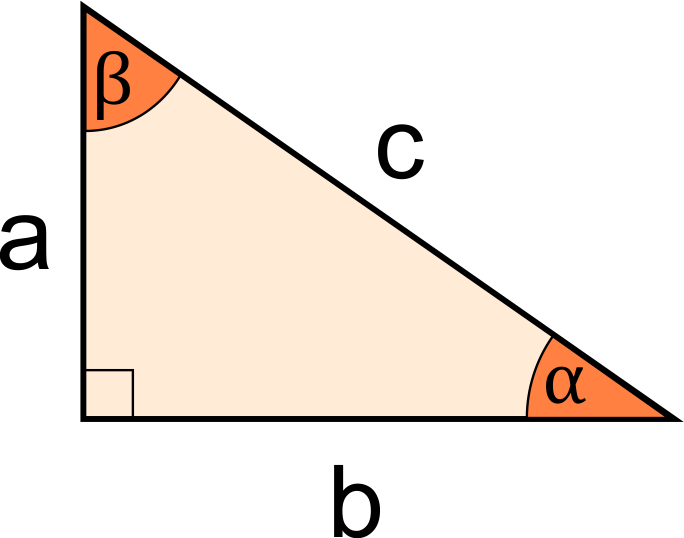# Check Similarity in Right Triangles Calculator

Reviewed by Rahul Dhari
Last updated: Jun 28, 2022

The check similarity in right triangles calculator enables you to identify if two right-angled triangles are similar, given their side lengths. Using this right triangles similarity checker, you can also find out if there is any reflection needed, to make the right triangles similar!

## When are right triangles similar?

Two right triangles will be similar if any of the following conditions are satisfied:

• The set of 3 angles in both triangles are the same; or
• The corresponding side lengths of the triangles are proportional. This proportionality constant is called the scale factor.

## How do I use the calculator to check similarity in right triangles?

Using this calculator to check similarity in right triangles involves only a few steps! You need to do the following:

1. Enter any 2 sides of the first right triangle in this right triangles similarity checker tool.
2. If you know the side lengths of only the 2 legs in the second triangle, enter them.
3. Alternatively, if you know all 3 side lengths of the second triangle, you may choose that option and enter all 3 sides.
4. Voila! The calculator to check similarity in right triangles will display a result showing whether or not the triangles are similar! Additionally, you will also be able to see if there are any reflections possible, that will make the triangles similar!

## Other similarity calculators

If you found this tool helpful, you may also find the following calculators useful:

## FAQ

### Is a 3-4-5 triangle similar to a 6-8-10 triangle?

Yes! A 6-8-10 triangle is similar to a 3-4-5 triangle because it is obtained by multiplying each side of the 3-4-5 triangle by 2! You may also verify it as follows:

1. Take the ratio of the sides 6 and 3, which would give us 2.
2. Find the ratio of sides 8 and 4, which would also give us 2.
3. Calculate the ratio of the final pair of sides (10 and 5), which would also lead us to 2.
4. Since all 3 pairs of sides are proportional with the same proportionality constant (2), a 3-4-5 triangle will be similar to a 6-8-10 triangle!

### Are 6, 8, and 10 a Pythagorean triplet?

Yes! 6, 8, and 10 form a Pythagorean triplet because the square of the largest side is equal to the sum of squares of the other 2 sides, which is the definition of the Pythagorean theorem. This can be verified as follows:

1. The square of the largest side (10) is 100.
2. The square of 6 is 36.
3. The square of 8 is 64.
4. Since 100 is equal to the sum of 36 and 64, these numbers (6, 8, and 10) form a Pythagorean triplet!
First triangle measurements (Enter any 2 sides)Length of a (leg)
in
Length of b (leg)
in
Length of c (hypotenuse)
in
Second triangle measurementsI know the value of
2 legs (a and b)
Length of a (leg)
in
Length of b (leg)
in
People also viewed…

### Isosceles triangle height

The isosceles triangle height calculator shows you the two heights of an isosceles triangle.

### Meat footprint

Check out the meat impact - on the environment and your health.

### Millionaire

This millionaire calculator will help you determine how long it will take for you to reach a 7-figure saving or any financial goal you have. You can use this calculator even if you are just starting to save or even if you already have savings.

### Subset

Use the subset calculator to generate the list of subsets of a given set or to determine how many subsets it has.